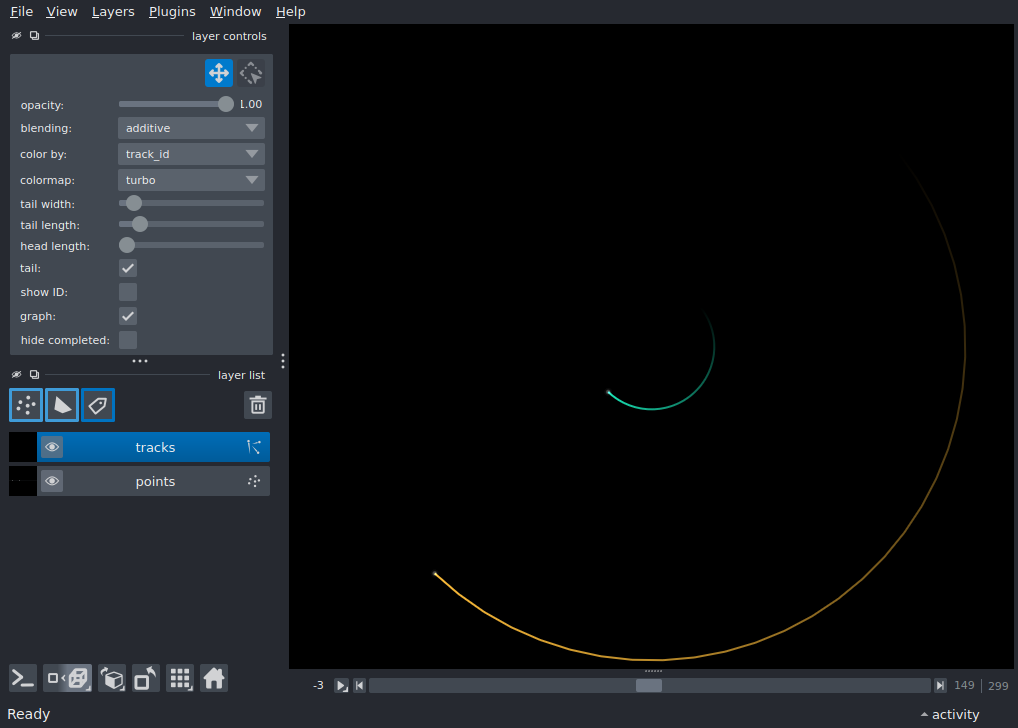# Tracks 3D with graph¶```import napari
import numpy as np

def _circle(r, theta):
x = r * np.cos(theta)
y = r * np.sin(theta)
return x, y

def tracks_3d_merge_split():
"""Create tracks with splitting and merging."""

timestamps = np.arange(300)

def _trajectory(t, r, track_id):
theta = t * 0.1
x, y = _circle(r, theta)
z = np.zeros(x.shape)
tid = np.ones(x.shape) * track_id
return np.stack([tid, t, z, y, x], axis=1)

trackA = _trajectory(timestamps[:100], 30.0, 0)
trackB = _trajectory(timestamps[100:200], 10.0, 1)
trackC = _trajectory(timestamps[100:200], 50.0, 2)
trackD = _trajectory(timestamps[200:], 30.0, 3)

data = [trackA, trackB, trackC, trackD]
tracks = np.concatenate(data, axis=0)
tracks[:, 2:] += 50.0  # centre the track at (50, 50, 50)

graph = {1: 0, 2: , 3: [1, 2]}

features = {'time': tracks[:, 1]}

return tracks, features, graph

tracks, features, graph = tracks_3d_merge_split()
vertices = tracks[:, 1:]

viewer = napari.Viewer(ndisplay=3)